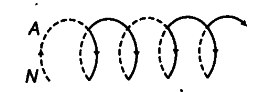# An observer to the left of a solenoid of N turns each of cross-section area

An observer to the left of a solenoid of N turns each of cross-section area A observes that a steady current I in it flows in the clockwise direction. Depict the magnetic field lines due to the solenoid specifying its polarity and show that it acts as a bar magnet of magnetic moment m = NIA.Since, it is given that the current flows in the clockwise direction for an observer on the left side of the solenoid.

It means that the left face of the solenoid acts as South pole and right face acts as North pole. Inside a bar, the magnetic field lines are directed from South to North.
Therefore, the magnetic field lines are directed from left to right in the solenoid.
Magnetic moment of a single current carrying loop is given by, m’ = IA.
So, magnetic moment of the whole solenoid is given by m = Nm’ = N(IA)